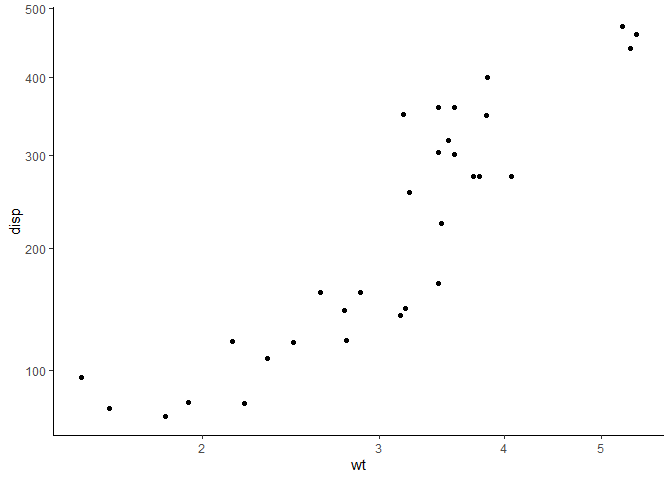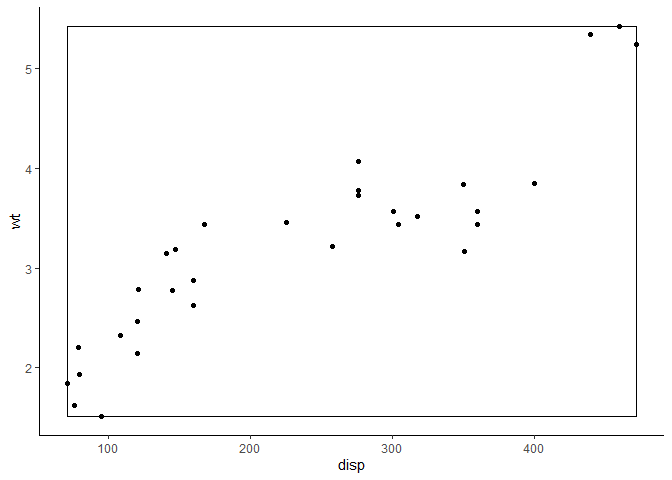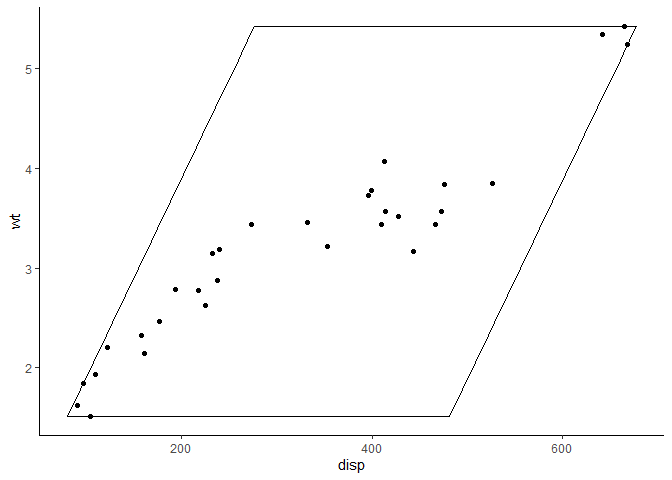# Transforming coordinate systems

{ggplot2} has many built-in coordinate systems which are used to both 1) produce the two-dimensional position of the plotted data and 2) draw custom axes and panel backgrounds. coord_geo() uses this second purpose to draw special axes that include timescales. However, deeptime also includes a number of other coordinate systems whose primary function is to modify the way data is plotted. To demonstrate this, we’ll first need to load some packages.

# Load deeptime
library(deeptime)
# Load ggplot for making plots
# It has some example data too
library(ggplot2)

## coord_trans meets coord_flip

One limitation of the traditional coord_trans() function in {ggplot2} is that you can not flip the axes while also transforming the axes. Historically, you would need to either 1) use scale_x_continuous() or scale_y_continuous() to transform one or both of your axes (which could result in the untransparent loss of data) in combination with coord_flip() or 2) transform your data before supplying it to ggplot(). coord_trans_flip() accomplishes this without the need for scales or transforming your data. It works just like coord_trans(), with the added functionality of the axis flip from coord_flip().

ggplot(mtcars, aes(disp, wt)) +
geom_point() +
coord_trans_flip(x = "sqrt", y = "log10") +
theme_classic()Note: back in 2020, {ggplot2} updated all the directional stats and geoms (e.g., boxplots and histograms) to work in both directions based on the aesthetic mapping. This somewhat makes this function redundant, but I still find it useful.

## 2D linear transformations

Another limitation of the traditional coord_trans() is that each axis is transformed independently. coord_trans_xy() expands this functionality to allow for a two-dimensional linear transformation as generated by ggforce::linear_trans(). This allows for rotations, stretches, shears, translations, and reflections. A dummy example using the ?mtcars dataset from {ggplot2} is included below. While applications of this functionality may seem abstract for real data, see the plotting traits article for a potential real-world application using species trait data.

# make transformer
library(ggforce)
trans <- linear_trans(shear(50, 0))

# set up data to be plotted
square <- data.frame(
disp = c(
min(mtcars$disp), min(mtcars$disp),
max(mtcars$disp), max(mtcars$disp)
),
wt = c(
min(mtcars$wt), max(mtcars$wt),
max(mtcars$wt), min(mtcars$wt)
)
)

# plot data normally
library(ggplot2)
ggplot(mtcars, aes(disp, wt)) +
geom_polygon(data = square, fill = NA, color = "black") +
geom_point(color = "black") +
coord_cartesian() +
theme_classic()# plot data with transformation
ggplot(mtcars, aes(disp, wt)) +
geom_polygon(data = square, fill = NA, color = "black") +
geom_point(color = "black") +
coord_trans_xy(trans = trans, expand = TRUE) +
theme_classic()